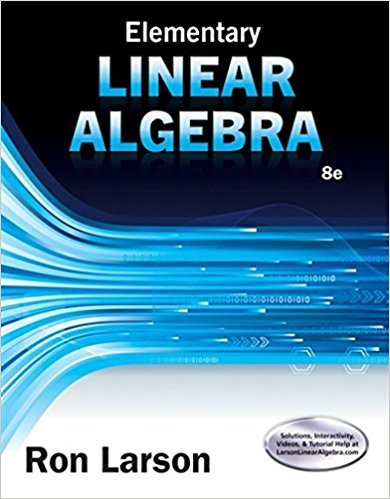×
×

# Subsets That Are Not Subspaces In Exercises 720, W is notISBN: 9781305658004 238

## Solution for problem 4.3.16 Chapter 4.3

Elementary Linear Algebra | 8th Edition

• Textbook Solutions
• 2901 Step-by-step solutions solved by professors and subject experts
• Get 24/7 help from StudySoup virtual teaching assistantsElementary Linear Algebra | 8th Edition

4 5 0 261 Reviews
18
0
Problem 4.3.16

Subsets That Are Not Subspaces In Exercises 720, W is not a subspace of the vector space. Verify this by giving a specific example that violates the test for a vector subspace (Theorem 4.5).W is the set of all matrices in M3,1 of the form[a 0 3a]T.

Step-by-Step Solution:
Step 1 of 3

\ I'rr f j! /'--1'rri t/ - r.'., f,)t J ',i'. - ,)...

Step 2 of 3

Step 3 of 3

##### ISBN: 9781305658004

This textbook survival guide was created for the textbook: Elementary Linear Algebra, edition: 8. Since the solution to 4.3.16 from 4.3 chapter was answered, more than 231 students have viewed the full step-by-step answer. The answer to “Subsets That Are Not Subspaces In Exercises 720, W is not a subspace of the vector space. Verify this by giving a specific example that violates the test for a vector subspace (Theorem 4.5).W is the set of all matrices in M3,1 of the form[a 0 3a]T.” is broken down into a number of easy to follow steps, and 47 words. Elementary Linear Algebra was written by and is associated to the ISBN: 9781305658004. This full solution covers the following key subjects: . This expansive textbook survival guide covers 45 chapters, and 6187 solutions. The full step-by-step solution to problem: 4.3.16 from chapter: 4.3 was answered by , our top Math solution expert on 01/12/18, 03:19PM.

Unlock Textbook Solution